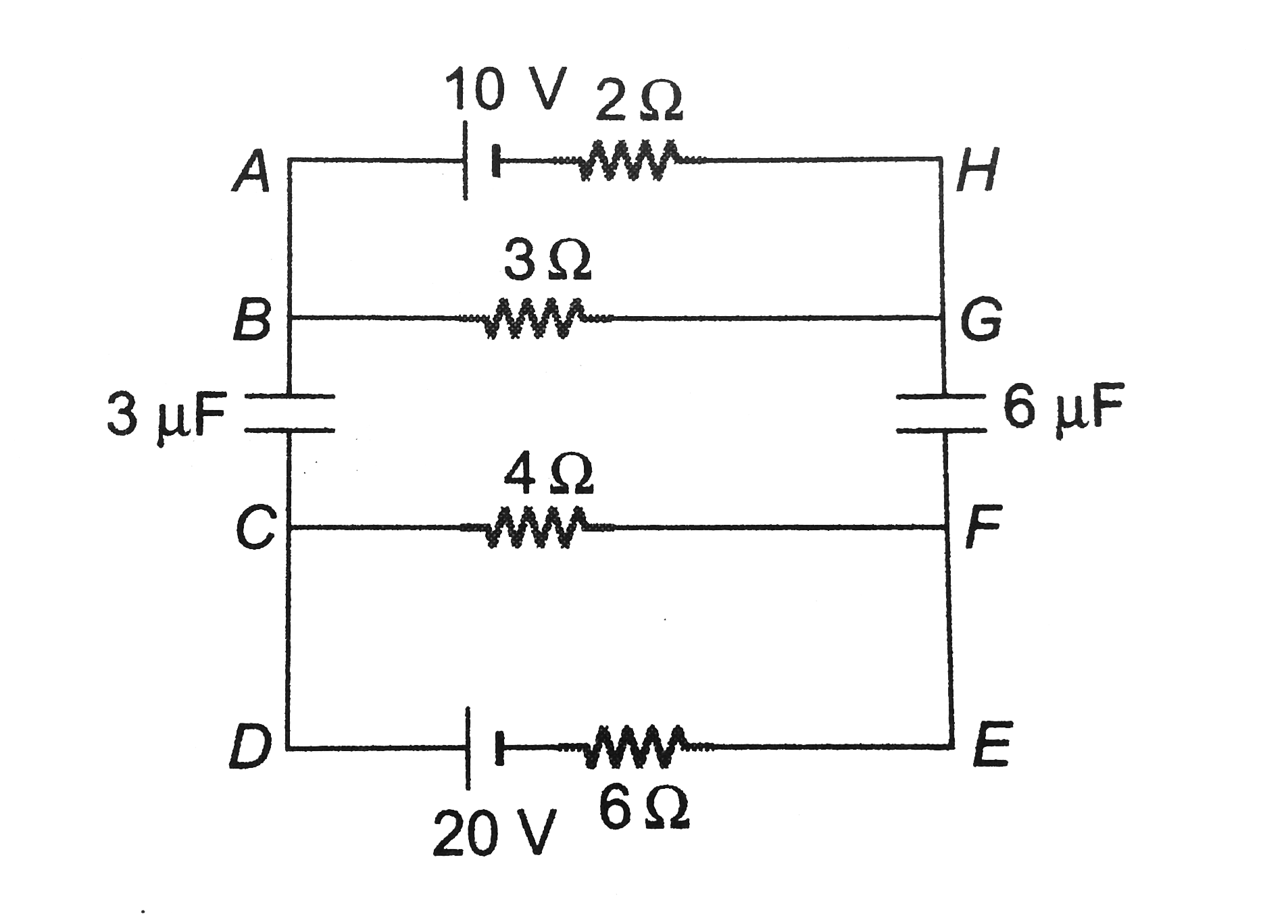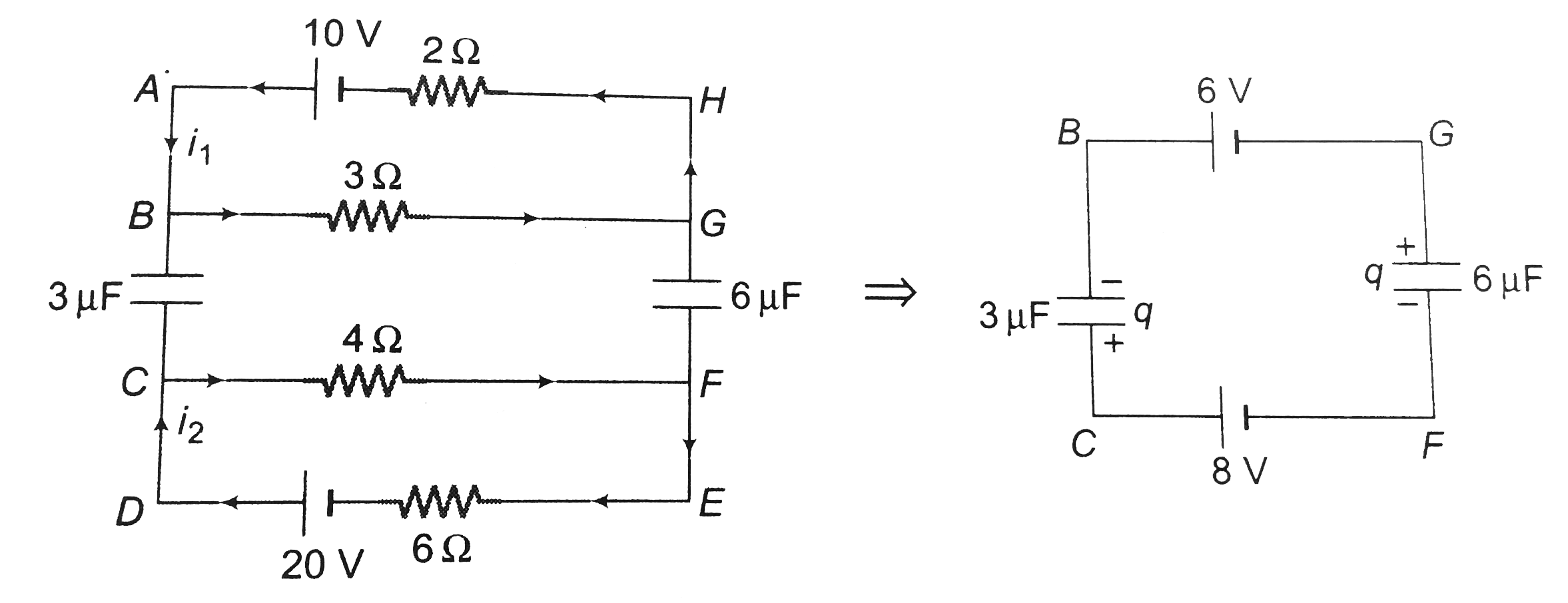Question

Updated on:26/04/2023

# In the circuit shown in figure, find the steady state charges on oth the capacitors.लिखित उत्तर

Solution
Current in the upper circuit i1=103+2=2A
VBG=VBVG=3i1=3×2=6V
current in the lower circuit
i2=204+6=2A
V(CF)=VCVF=4i2=4×2=8V
Charge on both the capacitors will be same. Let it be q. Applying Kirchoff's second law in loop BGFCB,=6a6×106+8q3×106=0
or (106)q2=2
orq=4×106C
orq=4μC